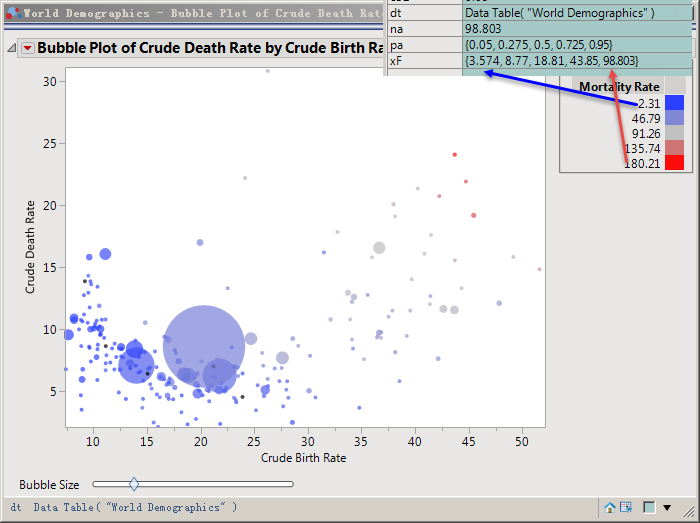Choose Language Hide Translation Bar
Highlighted

## How do I replace auto-generated parameters with custom color parameters in a Bubble Plot?

Dt = Open (" \$SAMPLE_DATA \ World Demographics. The JMP ");
Hide the first row and delete the first five columns with "(1000)" in the column name.
Generate the Bubble Plot with the following code.

``````dt = Current Data Table();
c11 = Quantile( 1, (:Crude Birth Rate << get values) ) * 1.01;
c12 = Quantile( 0, (:Crude Birth Rate << get values) ) * 0.99;
c21 = Quantile( 1, (:Crude Death Rate << get values) ) * 1.01;
c22 = Quantile( 0, (:Crude Death Rate << get values) ) * 0.99;
c31 = Quantile( 1, (:Population << get values) );
c32 = Quantile( 0, (:Population << get values) );
pa = {0.05, 0.275, 0.5, 0.725, 0.95};
xF = {};
For( c = 1, c <= 5, c++,
na = Quantile( pa[c], (:Infant Mortality Rate << get values) );
Insert Into( xF, na );
);

Bubble Plot(
X( :Crude Birth Rate ),
Y( :Crude Death Rate ),
Sizes( :Population ),
Coloring( :Infant Mortality Rate ),
ID( :Territory ),
Color Levels( xF ),
Title Position( 0, 0 ),
SendToReport(
Dispatch( {}, "1", ScaleBox, {Format( "Best", 10 ), Min( c12 ), Max( c11 ), Inc( 5 ), Minor Ticks( 1 )} ),
Dispatch( {}, "2", ScaleBox, {Format( "Best", 12 ), Min( c22 ), Max( c21 ), Inc( 5 ), Minor Ticks( 0 )} )
)
);
Wait( 0 );``````

How do I replace auto-generated parameters with custom color parameters in a Bubble Plot?
The parameter JSL code automatically generated by the software is

``Color Levels([2.31 46.785 91.26 135.735 180.21])``

How do I write JSL if I want to use my own parameters instead?Thanks!

1 ACCEPTED SOLUTION

Accepted Solutions
Highlighted

## Re: How do I replace auto-generated parameters with custom color parameters in a Bubble Plot?

The JMP Scripting Guide has a good overview of using matrices in JSL.  If you already have a list, you can convert it to a matrix:

``````Create a column vector from a single list:

A = Matrix( {1, 2, 3} );
[1,2,3]``````

You can also create an empty matrix and build it up by concatenation, similar to how you were using the list:

``````cl = [];
cl = cl || 100000;
cl = cl || 150000000;
cl = cl || 300000000;
cl = cl || 450000000;
cl = cl || 600000000;

[100000 150000000 300000000 450000000 600000000]``````

When you know the size of the matrix ahead of time, you can create the full matrix and use subscripts to assign values:

``````cl = J(1,5);
cl = 100000;
cl = 150000000;
cl = 300000000;
cl = 450000000;
cl = 600000000;
Show(cl);

cl = [100000 150000000 300000000 450000000 600000000];``````

Hope that helps!

3 REPLIES 3
Highlighted

## Re: How do I replace auto-generated parameters with custom color parameters in a Bubble Plot?

The argument to Color Levels() must be a matrix - the list argument will be ignored.  Here's an example that works:

``````Names Default To Here( 1 );
dt = Open( "\$SAMPLE_DATA/PopAgeGroup.jmp" );
cl = [100000 150000000 300000000 450000000 600000000];
obj = Bubble Plot(
X( :Name( "Portion 0-19" ) ),
Y( :Name( "Portion60+" ) ),
Sizes( :Pop ),
Coloring( :Pop ),
ID( :Country ),
Color Levels( cl )
);``````
Highlighted

## Re: How do I replace auto-generated parameters with custom color parameters in a Bubble Plot?

Thank danschikore!
Now the focus is on how to change xf into a matrix form, and I've searched and still can't find the answer.Ask for help.

I tried to write code like this, but it didn't work。Thanks!

``……Mat = Number Col Box("",xf) << get as matrix;……Color Levels( Mat ),``
Highlighted

## Re: How do I replace auto-generated parameters with custom color parameters in a Bubble Plot?

The JMP Scripting Guide has a good overview of using matrices in JSL.  If you already have a list, you can convert it to a matrix:

``````Create a column vector from a single list:

A = Matrix( {1, 2, 3} );
[1,2,3]``````

You can also create an empty matrix and build it up by concatenation, similar to how you were using the list:

``````cl = [];
cl = cl || 100000;
cl = cl || 150000000;
cl = cl || 300000000;
cl = cl || 450000000;
cl = cl || 600000000;

[100000 150000000 300000000 450000000 600000000]``````

When you know the size of the matrix ahead of time, you can create the full matrix and use subscripts to assign values:

``````cl = J(1,5);
cl = 100000;
cl = 150000000;
cl = 300000000;
cl = 450000000;
cl = 600000000;
Show(cl);

cl = [100000 150000000 300000000 450000000 600000000];``````

Hope that helps!

Article Labels

There are no labels assigned to this post.